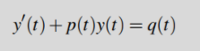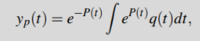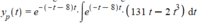# Question regarding finding the particular solution to nonhomogenous differentialequation.

#### tradBohus

##### New member
I'm having an issue with the integration in the following problem, perhaps you can help:
I'm meant to find the particular solution to the differential equation
y'(t)+(-2t-8)*y(t)=131t-2t3
, which is in the formI know that I can find the solution with the formula.
I have inserted P(t) (antiderivative of p(t)) and q(t) in the formula:However, I do not know how to approach the integration of eP(T)*q(t). Does anyone have any advice?Thank you.

#### tradBohus

##### New member
The solution is meant to be a second degree polynomial.